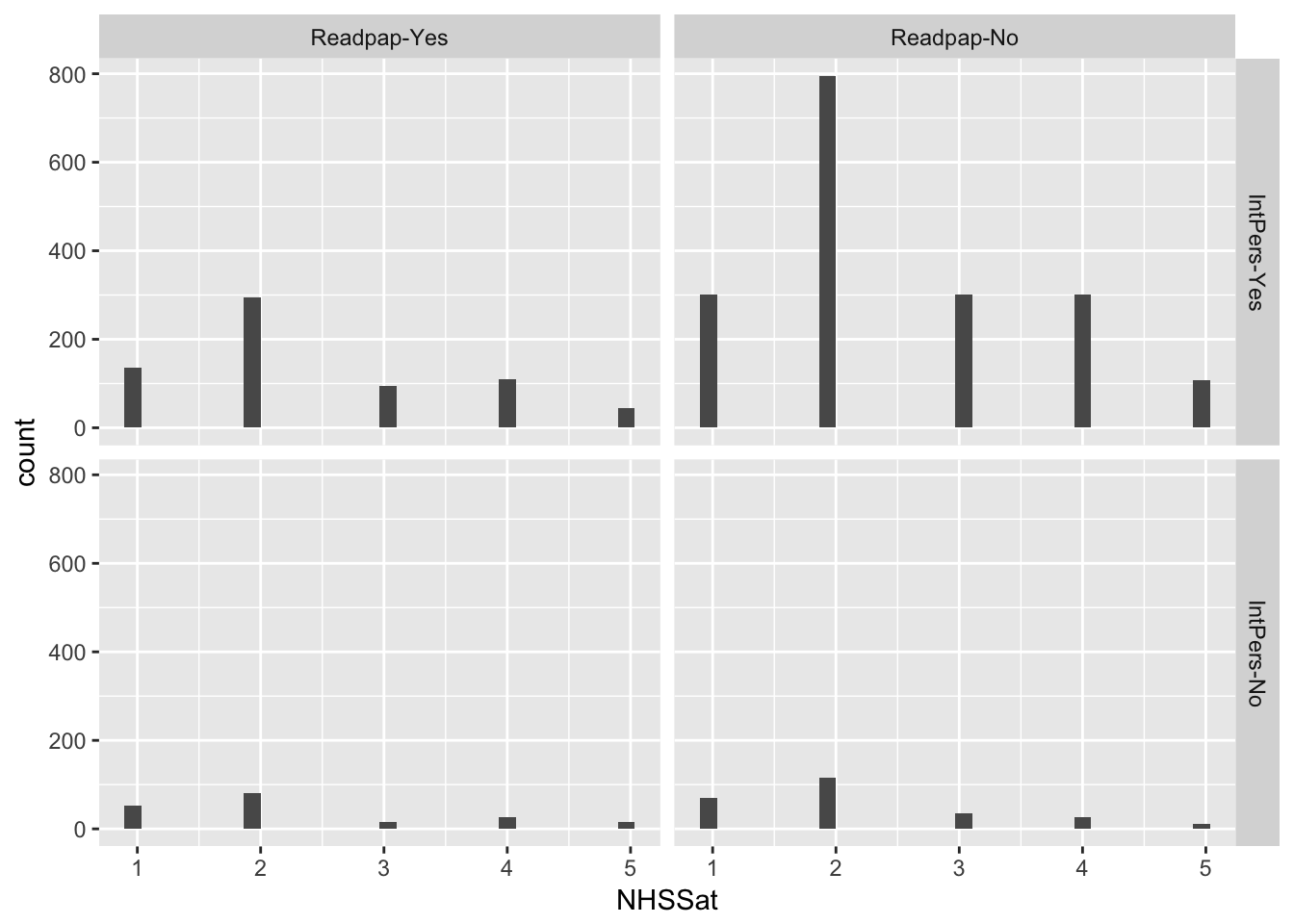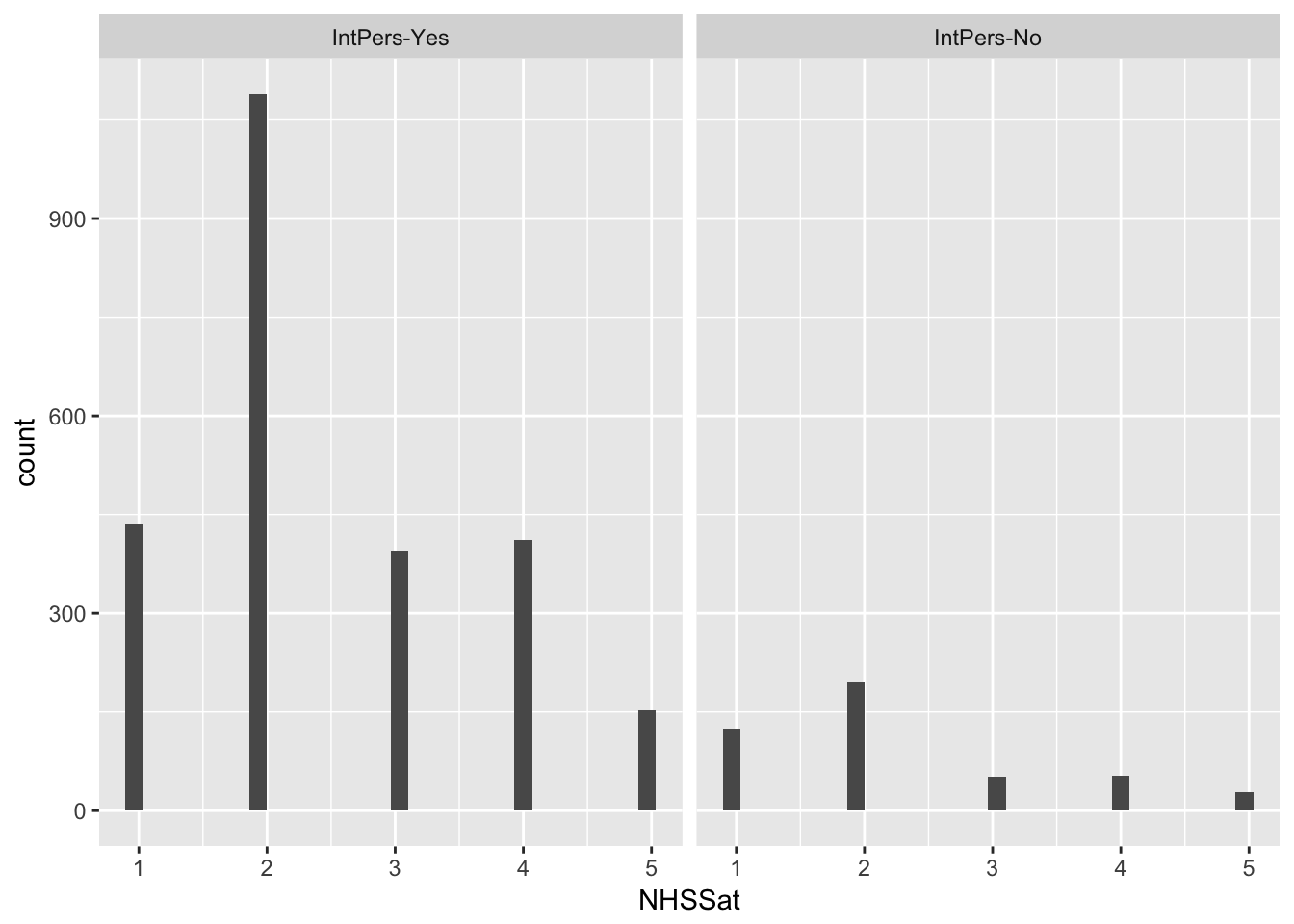# BSA

The code below loads the BSA data. Then we look at two example models. These are examples. Feel free to add your own.

rm(list=ls())
d <- read.table(file = 'bsa16_to_ukda.tab', header = TRUE, sep = '\t')

## Multiple regression

Let’s look at the questions in the documentation.

[Readpap] Do you normally read any daily morning newspaper at least 3 times a week? 1 Yes 2 No 8 (Don’t know) 9 (Refusal)

[IntPers] Do you personally have access to the internet, either at home, at work, or elsewhere, or on a smartphone, tablet or other mobile device? 1 Yes 2 No 8 (Don’t know) 9 (Refusal)

[NHSSat] CARD C1 All in all, how satisfied or dissatisfied would you say you are with the way in which the National Health Service runs nowadays? Choose a phrase from this card. 1 Very satisfied 2 Quite satisfied 3 Neither satisfied nor dissatisfied 4 Quite dissatisfied 5 Very dissatisfied 8 (Don’t know) 9 (Refusal)

d.1 <- d[d$Readpap < 8,] d.1 <- d.1[d.1$IntPers < 8,]
d.1 <- d.1[d.1$NHSSat < 8,] d.2 <- d.1[,c('Readpap', 'IntPers', 'NHSSat')] d.2$recode.Readpap <- factor(x = d.2$Readpap, labels = c('Readpap-Yes', 'Readpap-No')) d.2$recode.IntPers <- factor(x = d.2$IntPers, labels = c('IntPers-Yes', 'IntPers-No')) table(d.2$recode.Readpap, d.2$recode.IntPers, d.2$NHSSat)
## , ,  = 1
##
##
##               IntPers-Yes IntPers-No
##
## , ,  = 2
##
##
##               IntPers-Yes IntPers-No
##
## , ,  = 3
##
##
##               IntPers-Yes IntPers-No
##
## , ,  = 4
##
##
##               IntPers-Yes IntPers-No
##
## , ,  = 5
##
##
##               IntPers-Yes IntPers-No
##   Readpap-No          108         12
require(ggplot2)
## Loading required package: ggplot2
ggplot(data = d.2, aes(x = NHSSat)) +
geom_histogram(bins = 30) +
facet_grid(recode.IntPers~recode.Readpap)ex1.m <- lm(NHSSat ~ IntPers + Readpap, data = d.2)
summary(ex1.m)
##
## Call:
## lm(formula = NHSSat ~ IntPers + Readpap, data = d.2)
##
## Residuals:
##     Min      1Q  Median      3Q     Max
## -1.5032 -0.5032 -0.4848  0.5152  2.7511
##
## Coefficients:
##             Estimate Std. Error t value Pr(>|t|)
## (Intercept)  2.70237    0.11266  23.987  < 2e-16 ***
## IntPers     -0.23595    0.05900  -3.999 6.51e-05 ***
## Readpap      0.01839    0.04660   0.395    0.693
## ---
## Signif. codes:  0 '***' 0.001 '**' 0.01 '*' 0.05 '.' 0.1 ' ' 1
##
## Residual standard error: 1.144 on 2933 degrees of freedom
## Multiple R-squared:  0.005688,   Adjusted R-squared:  0.00501
## F-statistic: 8.389 on 2 and 2933 DF,  p-value: 0.0002328
ex2.m <- lm(NHSSat ~ IntPers + Readpap + IntPers * Readpap, data = d.2)
summary(ex2.m)
##
## Call:
## lm(formula = NHSSat ~ IntPers + Readpap + IntPers * Readpap,
##     data = d.2)
##
## Residuals:
##     Min      1Q  Median      3Q     Max
## -1.5127 -0.5127 -0.4594  0.5406  2.7992
##
## Coefficients:
##                 Estimate Std. Error t value Pr(>|t|)
## (Intercept)      2.33576    0.25716   9.083   <2e-16 ***
## IntPers          0.07027    0.20191   0.348    0.728
## Readpap          0.24445    0.14998   1.630    0.103
## IntPers:Readpap -0.19111    0.12051  -1.586    0.113
## ---
## Signif. codes:  0 '***' 0.001 '**' 0.01 '*' 0.05 '.' 0.1 ' ' 1
##
## Residual standard error: 1.144 on 2932 degrees of freedom
## Multiple R-squared:  0.00654,    Adjusted R-squared:  0.005524
## F-statistic: 6.434 on 3 and 2932 DF,  p-value: 0.0002436
AIC(ex1.m)
##  9127.86
AIC(ex2.m)
##  9127.343
ggplot(data = d.2, aes(x = NHSSat)) +
geom_histogram(bins = 30) +
facet_grid(~recode.IntPers)What do you think this means? How would you interpret these results?

## Multiple logistic regression

What about if we turn one of these predictors into our outcome? Can reading newspapers and satisfaction with the NHS explain much of the variance in internet access? I have intentionally picked a possibly senseless analysis as you should consider the question asked by your analysis carefully.

Let’s try this model out anyway.

As IntPers is 1 or 2, we should change this to 0 and 1. As 1 is yes, we will change 2 to 0 - so no is 0. Then we will fit the model.

d.2$logistic.IntPers <- d.2$IntPers
d.2$logistic.IntPers[d.2$logistic.IntPers == 2] <- 0
ex2.m <- glm(formula = logistic.IntPers ~ NHSSat + Readpap, family = binomial, data = d.2)
summary(ex2.m)
##
## Call:
## glm(formula = logistic.IntPers ~ NHSSat + Readpap, family = binomial,
##     data = d.2)
##
## Deviance Residuals:
##     Min       1Q   Median       3Q      Max
## -2.2438   0.4486   0.5352   0.5837   0.7897
##
## Coefficients:
##             Estimate Std. Error z value Pr(>|z|)
## (Intercept)  0.13999    0.20734   0.675      0.5
## NHSSat       0.18746    0.04742   3.953 7.72e-05 ***
## Readpap      0.67793    0.10570   6.414 1.42e-10 ***
## ---
## Signif. codes:  0 '***' 0.001 '**' 0.01 '*' 0.05 '.' 0.1 ' ' 1
##
## (Dispersion parameter for binomial family taken to be 1)
##
##     Null deviance: 2518.6  on 2935  degrees of freedom
## Residual deviance: 2461.5  on 2933  degrees of freedom
## AIC: 2467.5
##
## Number of Fisher Scoring iterations: 4

Both seem to be significant predictors of if a person responds yes to a question about if they have internet access.

# Over to you

The above are simple models. They show how you can use multiple linear and logistic regressions with the BSA data. Our choice of variables is perhaps questionable. Can you do any better? What sort of hypothesis can you investigate with the BSA data set?

Some points to consider:

• Sample sizes
• Does the data match test assumptions?
• Which variables to include?